## A Solution to the 4D Pyraminx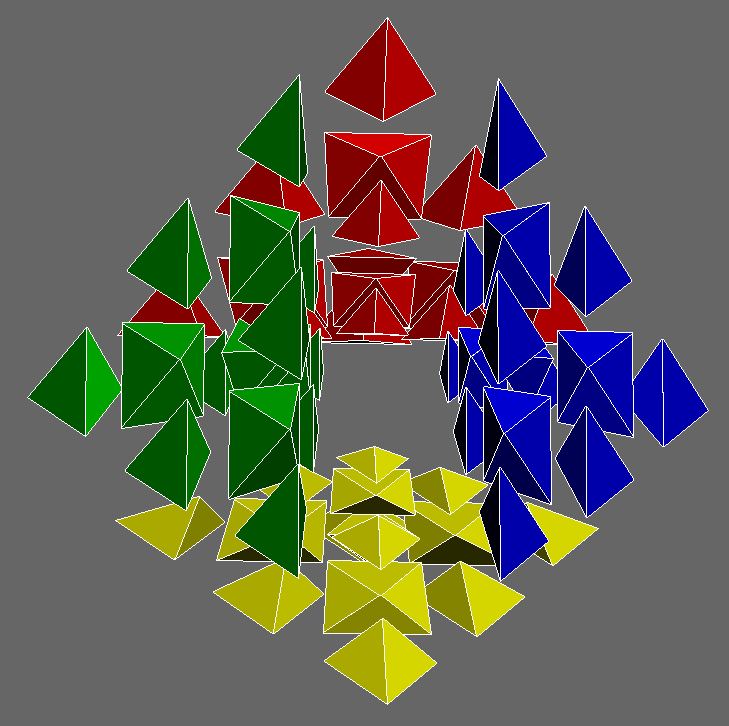The 4D pyraminx is the four-dimensional analog of the regular WCA-approved 3D pyraminx. This tutorial assumes you already know how to solve a 3D pyraminx.

### The Strategy

The 4D pyraminx can be solved in a way similar to the 3D pyraminx:

1. Solve the trivial tips and centers
2. Solve the edges

Using this approach, a solution of under 100 moves is easily achievable.

The puzzle is in MC4D, and can be accessed under “Puzzle” » “{3,3,3} Simplex” » “3”.

To rotate the puzzle in 4D, change the rotation style to “rotate by cubie”, and then control-click a cubie to rotate that cubie to the center. Also, drag the “Eye W Scale” slider all the way to the left. With this setting, centering the puzzle on a cell will reveal all five cells.

To turn a cell, left-click or right-click a cell’s sticker. For this puzzle, every vertex is opposite to some cell. Thus, to turn a vertex, we can click on a sticker on the opposite cell while holding down the “2” and/or “3” keys on the keyboard.

There are two views that we can use for this puzzle: vertex-first, and cell-first. The vertex-first view places a vertex in the middle, as follows:The cell-first view places a cell in the middle, as follows: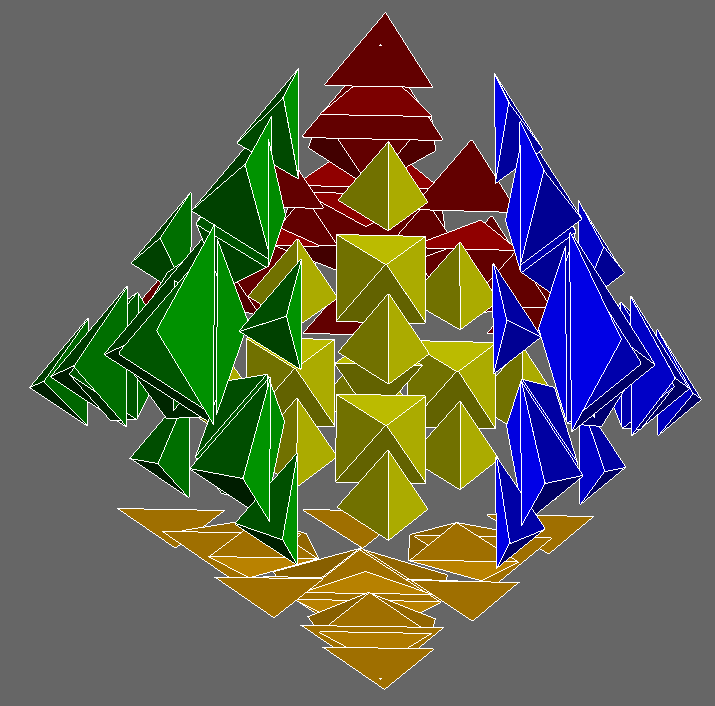The cell-first view is more confusing, due to the outer cells being inverted. This view does not have to be used for the solve.

### The Tips and Centers

To solve a tip or center, we first have to see where it belongs relative to the true centers. Unlike a regular pyraminx, where there is no fixed center, the 4D pyraminx provides us with one “true center” per cell, which indicates the orientation of the tips and centers. The next picture highlights 4 of the 5 true centers: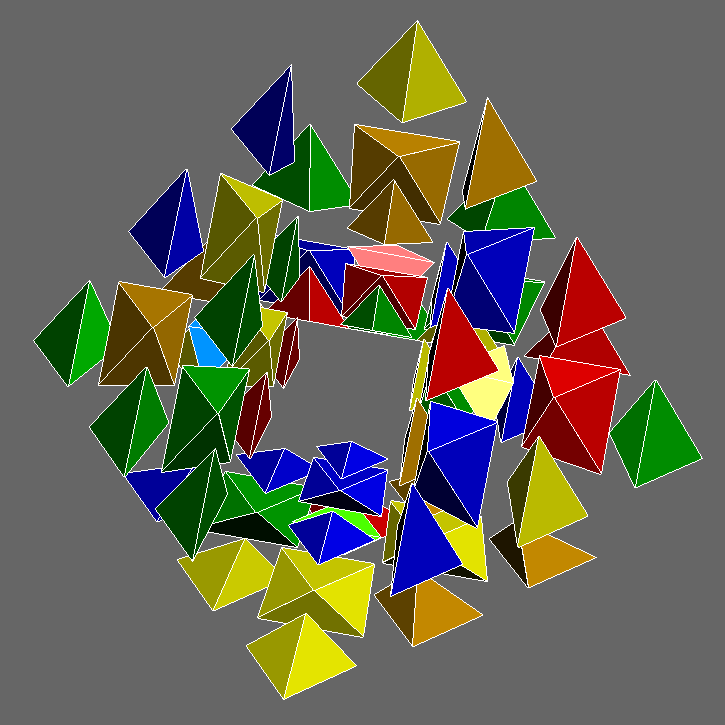Note that all four outer tips can be solved in at most one turn, each. All four outer centers can also be solved in at most one turn, each.

For any outer vertex, any clickable sticker on the cell opposite to that vertex can be clicked while holding down the “3” and/or “2” keys, in order to turn the tip and/or center, respectively.

Since there are five vertices, after solving the outer four vertices, we can rotate the puzzle in 4D to displace the central vertex. Control-clicking any outer vertex works, since that vertex goes to the center of the puzzle, displacing the central vertex. The central vertex then displaces a former outer vertex, and can now be solved.

When all the tips and centers are solved, the puzzle should look like the following: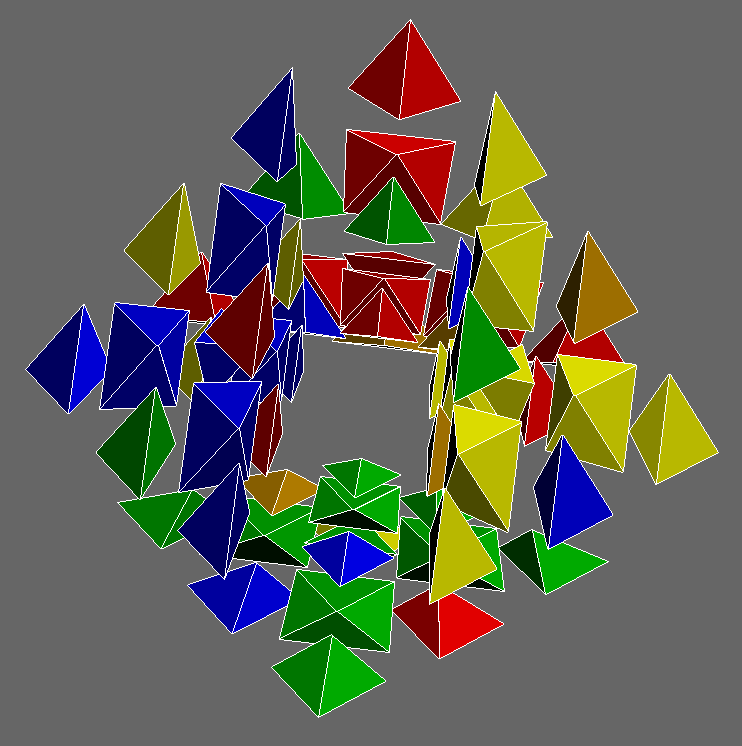### The Edges

To solve the edges, it turns out we can just use regular pyraminx algorithms such as the sledgehammer (R’ L R L’) or sexy move (R U R’ U’), along with their mirrors and inverses. There are 10 edges to solve in total, rather than 6.

To help speed up this step, setup moves and/or rotations can be used to place pieces more efficiently. The first layer does not have to be solved first. This step can be completed using just the vertex-first view.

However, we can opt to switch to a cell-first view for pieces that are already on a cell and oriented correctly, but permuted incorrectly: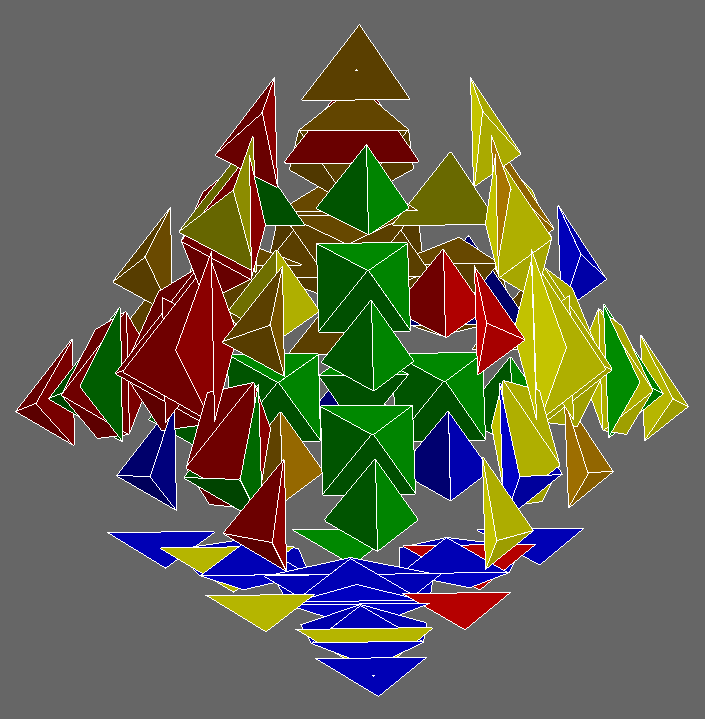In this example, the green-red-orange edge is oriented correctly on the green layer, but permuted incorrectly. Rather than resting between the green, red, and orange centers, the edge rests between the green, red, and yellow centers.

An anti-sune algorithm will solve the edge, as demonstrated by the arrows: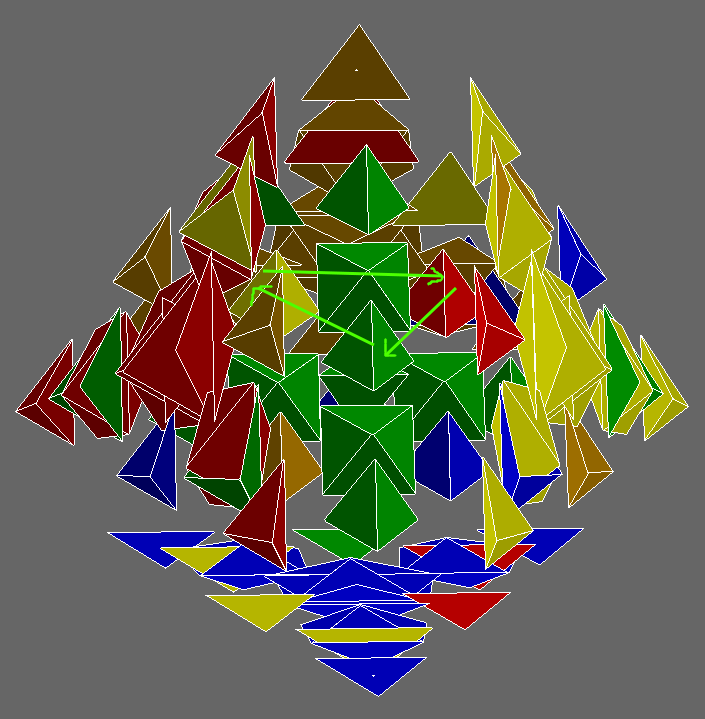### The Last Edge

Unlike the 3D pyraminx, it is possible for one edge to remain unsolved.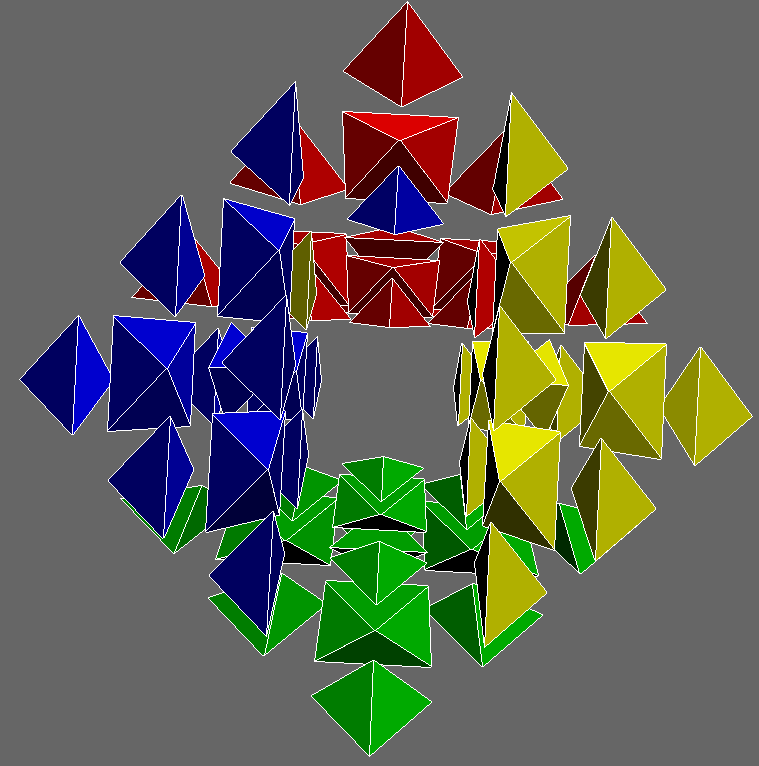A hint is that the commutator [sledgehammer, edge twist] flips two edges. Thus, one can use this algorithm to first flip the twisted edge and a solved edge, resulting in a case where two edges are permuted correctly, but have only 1 of 3 stickers solved. Then, the puzzle can be solved using that algorithm again, along with some setup moves.

Once you solve the puzzle, make an account on the Superliminal Wiki and note down your name on the solver’s list here, if you wish. If the wiki is down, feel free to make a post in the hypercubing Google group about your solve instead.

Go back to Articles